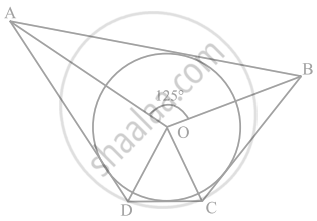# In figure, if ∠AOB = 125°, then ∠COD is equal to ______. - Mathematics

MCQ
Fill in the Blanks

In figure, if ∠AOB = 125°, then ∠COD is equal to ______.• 62.5°

• 45°

• 35°

• 55°

#### Solution

In figure, if ∠AOB = 125°, then ∠COD is equal to 55°.Explanation:

As in the given figure ABCD is a quadrilateral circumscribing the circle and we know that, the opposite sides of a quadrilateral circumscribing a circle subtend supplementary angles at the center of the circle.

So, we have

∠AOB + ∠COD = 180°

125° + ∠COD = 180°

∠COD = 55°

Concept: Tangent to a Circle
Is there an error in this question or solution?

#### APPEARS IN

NCERT Mathematics Exemplar Class 10
Chapter 9 Circles
Exercise 9.1 | Q 2 | Page 102
Share